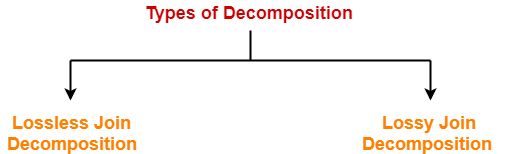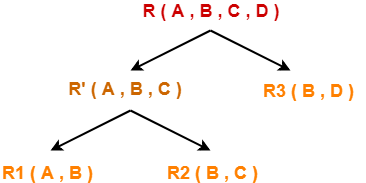# Determine Decomposition Is Lossless Or Lossy

## Decomposition in DBMS-

Before you go through this article, make sure that you have gone through the previous article on Decomposition in DBMS.

We have discussed-

• Decomposition is a process of dividing a single relation into two or more sub relations.
• Decomposition of a relation can be completed in the following two ways-1. Lossless Join Decomposition
2. Lossy Join Decomposition

In this article, we will learn how to determine whether the decomposition is lossless or lossy.

## Determining Whether Decomposition Is Lossless Or Lossy-

Consider a relation R is decomposed into two sub relations R1 and R2.

Then,

• If all the following conditions satisfy, then the decomposition is lossless.
• If any of these conditions fail, then the decomposition is lossy.

## Condition-01:

Union of both the sub relations must contain all the attributes that are present in the original relation R.

Thus,

 R1 ∪ R2 = R

## Condition-02:

• Intersection of both the sub relations must not be null.
• In other words, there must be some common attribute which is present in both the sub relations.

Thus,

 R1 ∩ R2 ≠ ∅

## Condition-03:

Intersection of both the sub relations must be a super key of either R1 or R2 or both.

Thus,

 R1 ∩ R2 = Super key of R1 or R2

## Problem-01:

Consider a relation schema R ( A , B , C , D ) with the functional dependencies A → B and C → D. Determine whether the decomposition of R into R1 ( A , B ) and R2 ( C , D ) is lossless or lossy.

## Solution-

To determine whether the decomposition is lossless or lossy,

• We will check all the conditions one by one.
• If any of the conditions fail, then the decomposition is lossy otherwise lossless.

## Condition-01:

According to condition-01, union of both the sub relations must contain all the attributes of relation R.

So, we have-

R1 ( A , B ) ∪ R2 ( C , D )

= R ( A , B , C , D )

Clearly, union of the sub relations contain all the attributes of relation R.

Thus, condition-01 satisfies.

## Condition-02:

According to condition-02, intersection of both the sub relations must not be null.

So, we have-

R1 ( A , B ) ∩ R2 ( C , D )

= Φ

Clearly, intersection of the sub relations is null.

So, condition-02 fails.

Thus, we conclude that the decomposition is lossy.

## Problem-02:

Consider a relation schema R ( A , B , C , D ) with the following functional dependencies-

A → B

B → C

C → D

D → B

Determine whether the decomposition of R into R( A , B ) , R2 ( B , C ) and R3 ( B , D ) is lossless or lossy.

## Solution-

### Strategy to Solve

When a given relation is decomposed into more than two sub relations, then-

• Consider any one possible ways in which the relation might have been decomposed into those sub relations.
• First, divide the given relation into two sub relations.
• Then, divide the sub relations according to the sub relations given in the question.

As a thumb rule, remember-

Any relation can be decomposed only into two sub relations at a time.

Consider the original relation R was decomposed into the given sub relations as shown-## Decomposition of R(A, B, C, D) into R'(A, B, C) and R3(B, D)-

To determine whether the decomposition is lossless or lossy,

• We will check all the conditions one by one.
• If any of the conditions fail, then the decomposition is lossy otherwise lossless.

## Condition-01:

According to condition-01, union of both the sub relations must contain all the attributes of relation R.

So, we have-

R ( A , B , C ) ∪ R3 ( B , D )

= R ( A , B , C , D )

Clearly, union of the sub relations contain all the attributes of relation R.

Thus, condition-01 satisfies.

## Condition-02:

According to condition-02, intersection of both the sub relations must not be null.

So, we have-

R ( A , B , C ) ∩ R3 ( B , D )

= B

Clearly, intersection of the sub relations is not null.

Thus, condition-02 satisfies.

## Condition-03:

According to condition-03, intersection of both the sub relations must be the super key of one of the two sub relations or both.

So, we have-

R ( A , B , C ) ∩ R3 ( B , D )

= B

Now, the closure of attribute B is-

B+ = { B , C , D }

Now, we see-

• Attribute ‘B’ can not determine attribute ‘A’ of sub relation R’.
• Thus, it is not a super key of the sub relation R’.
• Attribute ‘B’ can determine all the attributes of sub relation R3.
• Thus, it is a super key of the sub relation R3.

Clearly, intersection of the sub relations is a super key of one of the sub relations.

So, condition-03 satisfies.

Thus, we conclude that the decomposition is lossless.

## Decomposition of R'(A, B, C) into R1(A, B) and R2(B, C)-

To determine whether the decomposition is lossless or lossy,

• We will check all the conditions one by one.
• If any of the conditions fail, then the decomposition is lossy otherwise lossless.

## Condition-01:

According to condition-01, union of both the sub relations must contain all the attributes of relation R’.

So, we have-

R( A , B ) ∪ R2 ( B , C )

= R’ ( A , B , C )

Clearly, union of the sub relations contain all the attributes of relation R’.

Thus, condition-01 satisfies.

### Condition-02:

According to condition-02, intersection of both the sub relations must not be null.

So, we have-

R1 ( A , B ) ∩ R2 ( B , C )

= B

Clearly, intersection of the sub relations is not null.

Thus, condition-02 satisfies.

## Condition-03:

According to condition-03, intersection of both the sub relations must be the super key of one of the two sub relations or both.

So, we have-

R1 ( A , B ) ∩ R2 ( B , C )

= B

Now, the closure of attribute B is-

B+ = { B , C , D }

Now, we see-

• Attribute ‘B’ can not determine attribute ‘A’ of sub relation R1.
• Thus, it is not a super key of the sub relation R1.
• Attribute ‘B’ can determine all the attributes of sub relation R2.
• Thus, it is a super key of the sub relation R2.

Clearly, intersection of the sub relations is a super key of one of the sub relations.

So, condition-03 satisfies.

Thus, we conclude that the decomposition is lossless.

### Conclusion-

Overall decomposition of relation R into sub relations R1, R2 and R3 is lossless.

Next Article- Introduction to Normal Forms

Get more notes and other study material of Database Management System (DBMS).

Watch video lectures by visiting our YouTube channel LearnVidFun.

SummaryArticle Name
Determine Decomposition Is Lossless Or Lossy
Description
To determine whether decomposition is lossless or lossy, we check 3 conditions. Lossless Decomposition occurs if all conditions satisfy. Lossy decomposition occurs if any one condition fails.
Author
Publisher Name
Gate Vidyalay
Publisher Logo